0
121

# Direction Questions for NMAT PDF:

Download Direction Questions for NMAT PDF. Top 10 very important Direction Questions for NMAT based on asked questions in previous exam papers.

Take NMAT mock test

Question 1: A person rode his bicycle Northwards, then turned left and rode one km and again turned left and rode 2km. He found himself exactly one km West of his starting point. How far did he ride Northwards initially?

a) 1 km

b) 2km

c) 3km

d) 4km

e) 5km

Question 2: Town D is towards East of town F. Town B is towards North of town D. Town H is towards South of town B. Towards which direction is town H from town F?

a) East

b) South-East

c) North-East

e) None of these

Question 3: Ashok started walking towards South. After walking 50 meters he took a right turn and walked 30 meters. He then took a right turn and walked 100 meters. He again took a right turn and walked 30 meters and stopped. How far and in which direction was he from the starting point?

a) 50 meters South

b) 150 meters North

c) 180 meters East

d) 50 meters North

e) None of these

Question 4: Point R is 10 metres north of point A. Point K is exactly in the middle of the points R and A. Point N is 7 metres east of point A. Point M is 7 metres east of point K. Point S is 6 metres north of point M. What is the distance between points S and N?

a) 13 metres

b) 16 metres

c) 11 metres

d) 12 metres

e) None of these

Question 5: Mohan walked 30 meters towards South, took a left turn and walked 15 meters. He then took a right turn and walked 20 meters. He again took a right turn and walked 15 meters.How far is he from the starting point ?

a) 95 meters

b) 50 meters

c) 70 meters

d) Cannot be determined

e) None of these

Question 6: Ashok started walking towards South. After walking 50 meters he took a right turn and walked 30 meters. He then took a right turn and walked 100 meters. He again took a right turn and walked 30 meters and stopped. How far and in which direction was he from the starting point ?

a) 50 meters South

b) 150 meters North

c) 180 meters East

d) 50 meters North

e) None of these

Question 7: Sita starts from point A and travels towards north for 2 km, then she takes a turn of 60° to her right and travels for another 2 km, then she takes a turn of 120° to her right and travels for another 2 km. Finally, she takes a turn of 135° to her left.
What is the direction she is finally facing?

a) North

b) South -west

c) North-east

d) East

e) Cannot be determined

Question 8: Karan starts from point A and travels towards north for 5 km. He then takes a right turn and travels for 7 km. He then takes a left turn and travels another 7 km. Finally he takes another left turn and travels for 2 km arriving at a point B.
What is the distance between point A and B?

a) 13 km

b) 12.33 km

c) 12 km

d) 10 km

e) None of these

Question 9: Anup starts from point A and travels towards north for 8 km and then he turns to his left and travels for 15 km to reach point B.
What is the distance between A and B and the direction to which Anup is finally facing?

a) 17 km, West.

b) 23 km, East.

c) 19 km, West.

d) 21 km, East.

e) None of these

Question 10: In a row of twenty-five children facing South R is sixteenth from the right end and B is eighteenth from the left end. How many children are there between R and B?

a) 2

b) 3

c) 4

e) None of these

According to the question, way of riding of the person is as follow.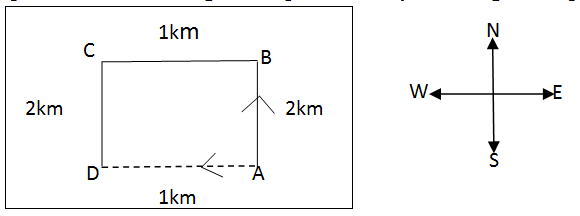As per the question, D is 1km West of A, therefore AB=DC=2km.

The below are the different possible positions of the cities:

B                     OR                   B                  OR                     B

F          H/D                                   F      D                                           H

H                           F               D

Hence H can be South-East of F or East of F or North-East.

Ashok walked 50m towards South. Then he took right and walked 30m=> he is travelling towards East. Now, he is 50m South and 30m East from his starting position.

Then he took right and travelled 100m => Now he is walking towards North and is 50m North and 30m East from his starting position.

Finally, he took right again and travelled 30m => He is walking towards West and is 50m North from his starting position.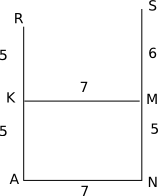The required distance = 11 m

The movement of Mohan is as shown in the diagram below. So, he is 30 + 20 = 50 metres from the original spot.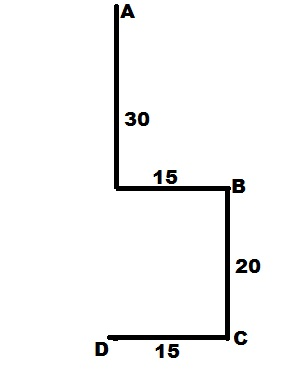The movement of Ashok is as shown below. So, he is North of the original spot by 100 – 50 = 50 metres.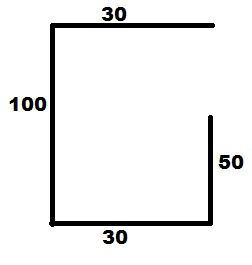Based on the given information, the path travelled by sita could be as below.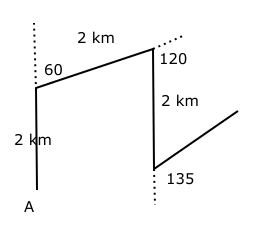Therefore the direction she finally faces is North-east.

Based on the given information, the path travelled by karan could be derived as below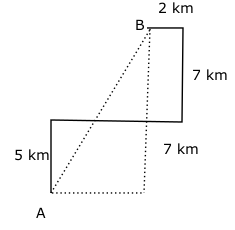Therefore the distance between A and B = $\sqrt{12^{2} + 5^{2}}$ = 13

So the correct option to choose is A – 13 km.

Based on the given information, the path travelled by Anup could be as below.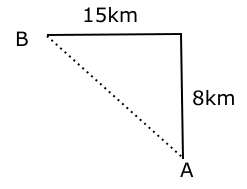Distance between A and B = $\sqrt{8^2 + 15^2}$ = 17 km.

Anup faces west at point B.

So the correct option to choose is A.

Total number of children in the row = 25

Position of R from right end = 16th

Position of B from left end = 18th

=> Position of B from right end = (25 – 18) + 1 = 8th

$\therefore$ Number of children between R and B = (16 – 8) – 1 = 7

=> Ans – (E)

We hope this Direction Questions for NMAT pdf for NMAT exam will be highly useful for your Preparation.